Online Tution   »   Class 10   »   Polynomial Class 10

# Polynomials- Degree, Definition, Zero Polynomial for Class 9 & 10

## Polynomials Definition

Exponents in an algebraic expression may be rational numbers. The exponent on any variable in an algebraic statement called a polynomial, on the other hand, must be a whole number.

Let us consider few more examples,

5×3+3x+1 is an example of a polynomial. It is an algebraic expression as well.

2x+3√x is an algebraic expression, but not a polynomial. – since the exponent on x is 1/2 which is not a whole number.

## What is Degree Of Polynomials?

The degree of the polynomial is the largest exponent on the variable in a polynomial with one variable.

Example: The polynomial x2+2x+3 has a degree of 2, as x2 is the largest power of x in the provided expression.

Another illustration: The polynomial x8 + 2×6 – 3x + 9 has degree 8 because that number is the highest power in the supplied expression.

## What is Types Of Polynomials?

Polynomials can be classified based on:

1. a) Number of terms
2. b) Degree of the polynomial.

## Types of polynomials based on the number of terms

1. a) Monomial – A polynomial with just one term. Example: 2x, 6×2, 9xy

1. b) Binomial – A polynomial with two unlike terms. Example: 4×2+x, 5x+4

1. c) Trinomial – A polynomial with three unlike terms. Example: x2+3x+4

### Linear Polynomial

A polynomial whose degree is one is called a linear polynomial.

For example, 2x+1 is a linear polynomial.

A polynomial of degree two is called a quadratic polynomial.

For example, 3×2+8x+5 is a quadratic polynomial.

### Cubic Polynomial

A polynomial of degree three is called a cubic polynomial.

For example, 2×3+5×2+9x+15 is a cubic polynomial.

## Polynomials for Class 9 and 10 Graphical Representation

Here, let’s examine the graph representation of a polynomial equation.

#### Equation Representation On A Graph

Every point on the graph represents the x and y coordinates of the point that satisfies the equation, and any equation may be represented as a graph on the Cartesian plane. Any point that fulfils an equation will reside on the curve since an equation is only a constraint on the x and y coordinates of a point.

For instance, the straight line formed by the graph’s equation y = x will connect all the spots whose x and y coordinates are equal. For instance, (1,1), (2,2), and so forth.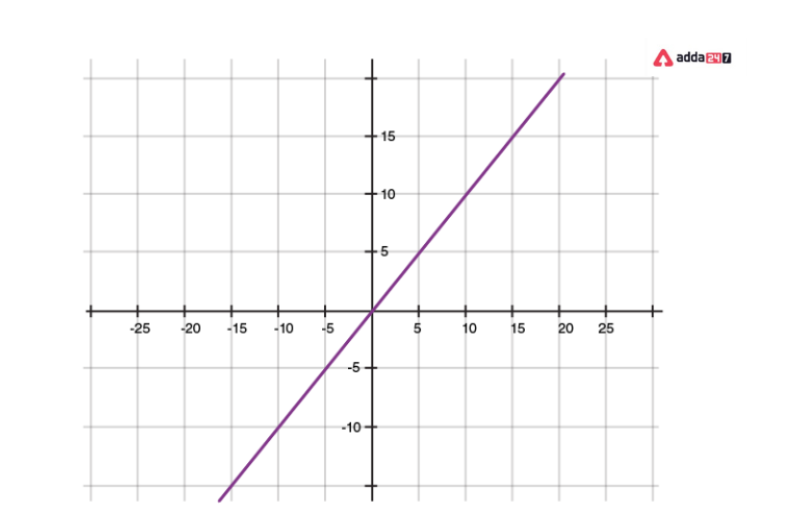## Geometrical Representation Of A Linear Polynomial

A linear polynomial has a straight line for its graph. It precisely cuts the X-axis at one place.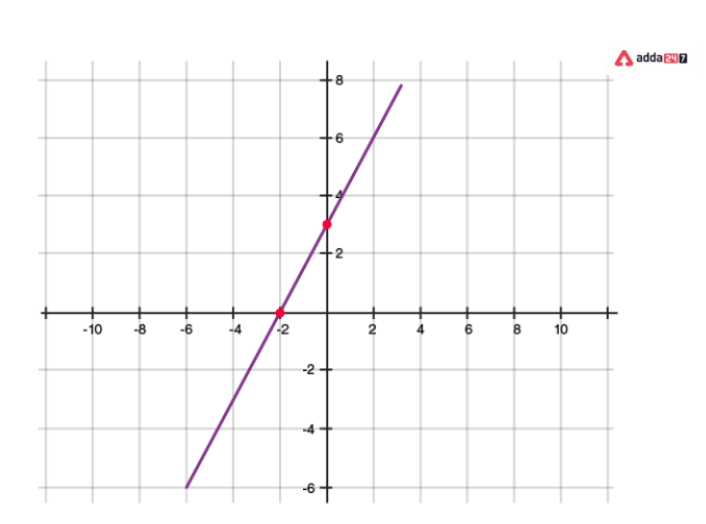## Geometrical Representation Of A Quadratic Polynomial

• A parabola is a quadratic polynomial’s graph.
• It resembles a U that, depending on the value of “a” in ax2+bx+c, either opens upwards or downwards.
• The parabola opens upwards if “a” is positive, and downwards if “a” is negative.
• The x-axis can be clipped at 0, 1, or two points.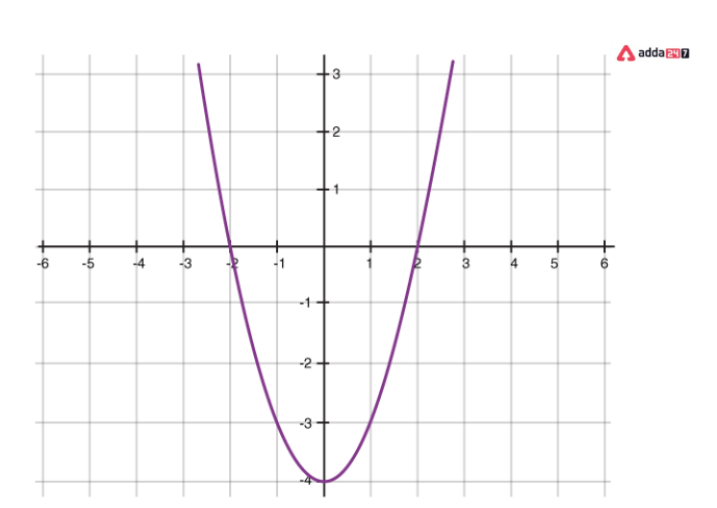Graph of a polynomial which cuts the x-axis in two distinct points (a>0)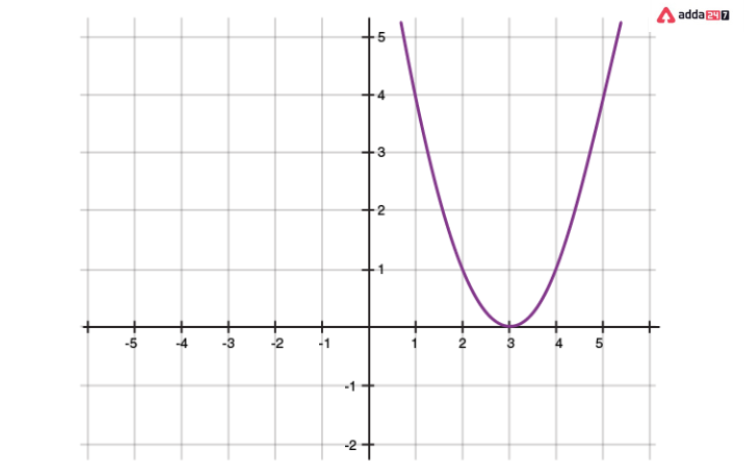A graph of a quadratic polynomial with one point touching the x-axis (a>0)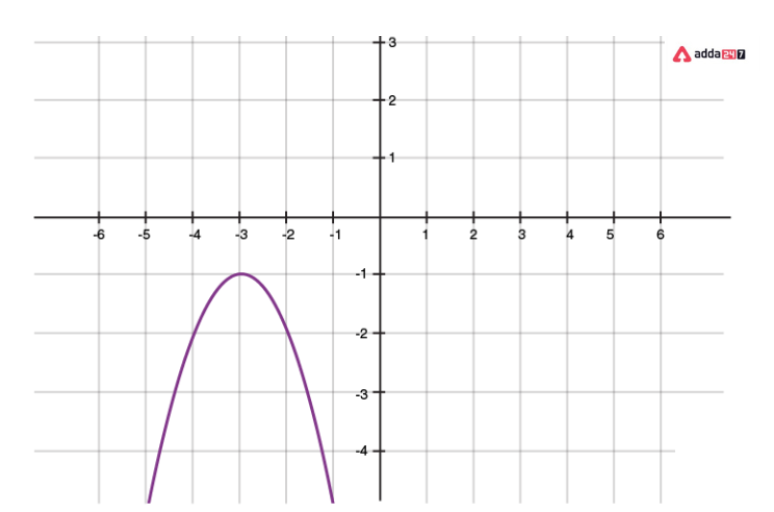A quadratic polynomial’s (a<0) graph showing that it does not touch the x-axis.

## Graph of the polynomial x^n

For a polynomial of the form y=xn where n is a whole number:

• as n increases, the graph becomes steeper or draws closer to the Y-axis
• If n is odd, the graph lies in the first and third quadrants
• If n is even, the graph lies in the first and second quadrants
• The graph of y=−xn is the reflection of the graph of y=xn on the x-axis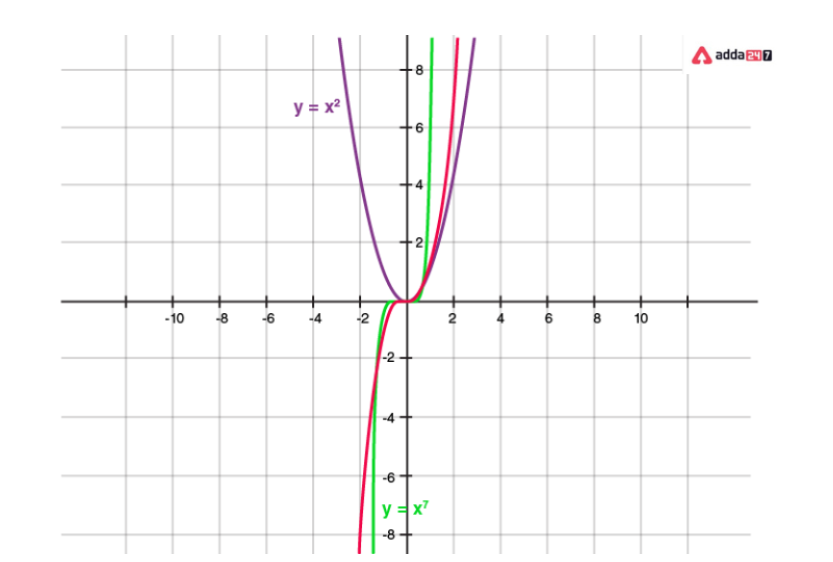Polynomial graph with various degrees.

## Zero Polynomial

A zero of a polynomial p(x) is the value of x for which the value of p(x) is 0. If k is a zero of p(x), then p(k)=0.

For example, consider a polynomial p(x)=x2−3x+2.

When x=1, the value of p(x) will be equal to

p(1)=12−3×1+2

=1−3+2

=0

Since p(x)=0 at x=1, we say that 1 is a zero of the polynomial x2−3x+2

## Geometrical Meaning Of Zeros Of A Polynomial

A polynomial’s zeros are, geometrically speaking, the locations where its graph intersects the x-axis.

(i) One zero (Linear Polynomial)    (ii) Two zeros (Quadratic Polynomial)    (iii) Three zeros (Cubic Polynomial)

Here A, B and C correspond to the zeros of the polynomial represented by the graphs.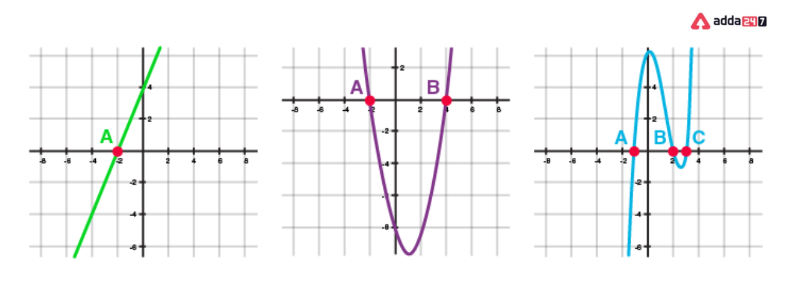## Number Of Zero Polynomial

A polynomial of degree n typically contains no more than n zeros.

• One zero exists in a linear polynomial.
• Maximum zeros in a quadratic polynomial are two.
• A cubic polynomial can only have three zero.

Ace your class 10th board exams with Adda247 live classes for class 10th preparation.

## Polynomials Factorisation

Quadratic polynomials can be factorized by splitting the middle term.

For example, consider the polynomial 2×2−5x+3

Splitting the middle term: The middle term in the polynomial 2×2−5x+3 is -5x. This must be expressed as a sum of two terms such that the product of their coefficients is equal to the product of 2 and 3 (coefficient of x2 and the constant term)

−5 can be expressed as (−2)+(−3), as −2×−3=6=2×3

Thus, 2×2−5x+3=2×2−2x−3x+3

Now, identify the common factors in individual groups

2×2−2x−3x+3=2x(x−1)−3(x−1)

Taking (x−1) as the common factor, this can be expressed as:

2x(x−1)−3(x−1)=(x−1)(2x−3)

## Polynomial Division Algorithm

Let us assume that P(x) and G(x) are the two polynomials, such that G(x)≠ 0, then the division algorithm states the formula to find Q(x) and R(x) of the polynomial.

Here, P(x) denotes dividend polynomial

G(x) denotes divisor polynomial

Q(x) denotes quotient polynomial

R(x) denotes the remainder polynomial.

Thus, the formula stated by division algorithm is:

P(x) = G(x) × Q(x) + R(x)

Follow the instructions below to divide one polynomial by another.

Step 1: First, sort the terms of the dividend and the divisor according to decreasing degree.

Step 2: Subtract the highest degree term of the dividend from the highest degree term of the divisor to get the first term of the quotient. execute the division process after that.

Step 3: The payout for the following division is the leftovers from the previous division. Continue doing this until the remainder’s degree is lower than the divisor’s degree.

## Polynomials Class 9 and 10 MCQ

Ques. If the sum of zeroes of the quadratic polynomial 3×2 – kx + 6 is 3, then find the value of k.

Solution: Here a = 3, b = -k, c = 6

Sum of the zeroes, (α + β) = −ba = 3 …..(given)

⇒ −(−k)3 = 3

⇒ k = 9

Ques. Find the condition that zeroes of polynomial p(x) = ax2 + bx + c are reciprocal of each other.

Solution: Let α and 1α be the zeroes of P(x).

P(a) = ax2 + bx + c …(given)

Product of zeroes = ca

⇒ α × 1α = ca

⇒ 1 = ca

⇒ a = c (Required condition)

Coefficient of x2 = Constant term

Ques. Find a quadratic polynomial, the sum and product of whose zeroes are -8 and 12 respectively. Hence find the zeroes.

Solution: Let Sum of zeroes (α + β) = S = -8 …[Given]

Product of zeroes (αβ) = P = 12 …[Given]

Quadratic polynomial is x2 – Sx + P

= x2 – (-8)x + 12

= x2 + 8x + 12

= x2 + 6x + 2x + 12

= x(x + 6) + 2(x + 6)

= (x + 2)(x + 6)

Zeroes are:

x + 2 = 0 or x + 6 = 0

x = -2 or x = -6

Ques. Can (x – 2) be the remainder on division of a polynomial p(x) by (2x + 3)? Justify your answer.

Solution: In case of division of a polynomial by another polynomial, the degree of the remainder (polynomial) is always less than that of the divisor. (x – 2) can not be the remainder when p(x) is divided by (2x + 3) as the degree is the same.

Ques. If p(x) = x3 – 2×2 + kx + 5 is divided by (x – 2), the remainder is 11. Find k. Hence find all the zeroes of x3 + kx2 + 3x + 1.

Solution: p(x) = x3 – 2×2 + kx + 5,

When x – 2,

p(2) = (2)3 – 2(2)2 + k(2) + 5

⇒ 11 = 8 – 8 + 2k + 5

⇒ 11 – 5 = 2k

⇒ 6 = 2k

⇒ k = 3

Let q(x) = x3 + kx2 + 3x + 1

= x3 + 3×2 + 3x + 1

= x3 + 1 + 3×2 + 3x

= (x)3 + (1)3 + 3x(x + 1)

= (x + 1)3

= (x + 1) (x + 1) (x + 1) …[∵ a3 + b3 + 3ab (a + b) = (a + b)3]

All zeroes are:

x + 1 = 0 ⇒ x = -1

x + 1 = 0 ⇒ x = -1

x + 1 = 0 ⇒ x = -1

Hence zeroes are -1, -1 and -1.

Let’s connect via chat or call our senior expert counsellor at +91-9625869989 to learn more about the different streams and options available.

We would love it if we could add some of your insights. If you have a definite goal of scoring the highest marks, then you can resolve your doubts via our app/quizzes and youtube class  assistance (https://youtube.com/c/Adda247Class9and10)

## Polynomial- FAQs

Ques. What does a polynomial class 10 zero mean?

Ans. The locations where a polynomial becomes zero overall are known as the zeros of the polynomial.

Ques. What is a polynomial’s value?

Ans. The value of a polynomial at a particular point is defined as the value that the provided function yielded at that moment.

Ques. Are roots present in all polynomials?

Ans. Any real number that is not negative has a square root, as does every polynomial with an odd degree and real coefficients.

Ques. Which polynomial’s initial term is each one?

Ans. The leading coefficient is the first term of a polynomial.

Ques.A polynomial might not have any zeros.

Ans. There is no zero in a quadratic polynomial.

Ques. Can there be more than one zero in a polynomial?

Ans. In a polynomial, there can only be one zero.

Ques. Can Polynomials may be infinite.

Ans. Of course it can. However, any polynomial of degree infinity has either infinite or no solutions.

Ques. Are binomials a type of polynomial?

Ans. Since polynomials can have more than two terms, they are not all binomials. The claim is untrue as a result.

Ques. What are the polynomial’s two components?

Ans. Simple rules govern the definition of polynomials. Because polynomials are sums of variables and exponents, the prefix “Poly” denotes “many.”

Sharing is caring!

Thank You, Your details have been submitted we will get back to you.
•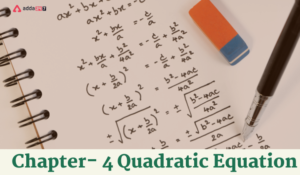Quadratic Equation Class 10 Chapter 4 No...
•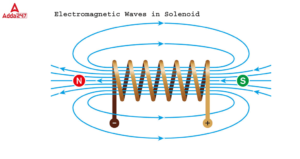What is a Solenoid?- Coil, Valve, Lock, ...
•Manufacturing Industries- CBSE Class 10 ...
•A Letter to God Summary, Questions and A...
•Class 10 English Sample Paper 2022- 23 w...
•Minerals and Energy Resources Class 10 N...